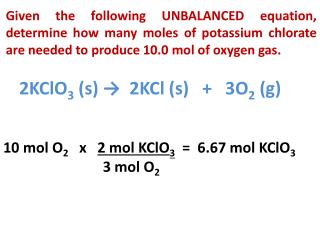Download Presentation2KClO 3 (s) → 2KCl (s) + 3O 2 (g)

# 2KClO 3 (s) → 2KCl (s) + 3O 2 (g)

Download Presentation## 2KClO 3 (s) → 2KCl (s) + 3O 2 (g)

- - - - - - - - - - - - - - - - - - - - - - - - - - - E N D - - - - - - - - - - - - - - - - - - - - - - - - - - -
##### Presentation Transcript

1. Given the following UNBALANCED equation, determine how many moles of potassium chlorate are needed to produce 10.0 mol of oxygen gas. 2KClO3 (s) → 2KCl (s) + 3O2 (g) 10 mol O2 x 2 mol KClO3 = 6.67 mol KClO3 3 mol O2

2. How many moles of oxygen gas are produced if 5.25 mol of potassium chlorate decomposes? 2KClO3 (s) → 2KCl (s) + 3O2 (g) 5.25 mol KClO3 x 3 mol O2 = 7.88 mol O2 2 mol KClO3

3. How many moles of potassium chloride are produced if 3.70 g of KClO3 is decomposed? 2KClO3 (s) → 2KCl (s) + 3O2 (g) 3.70 g KClO3 x 1 mol KClO3 x 2 mol KCl = 0.0302 mol KCl 122.5 g KClO32 mol KClO3

4. How many grams of O2 are produced if 2.50 g of KClO3 is decomposed? 2KClO3 (s) → 2KCl (s) + 3O2 (g) 2.5 g KClO3 x 1 mol KClO3 x 3 mol O2 x 32.0 g O2 = 122.5 g KClO3 2 mol KClO3 1 mol O2 = 0.980 g O2

5. How many moles of water are produced when 20.0 g of hydrazine is reacted with 30.0 g of oxygen gas? N2H4 (l) + O2 (g) → N2 (g) + 2H2O (g) 20.0 g N2H4 x 1 mol N2H4x 2 mol H2O= 1.23 mol H2O 32.06 g N2H4 1 mol N2H4 30.0 g O2 x 1 mol O2x 2 mol H2O= 1.88 mol H2O 32.00 g O21 mol O2